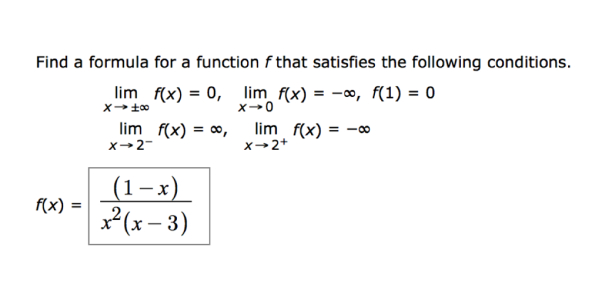Which of the following can be true if function f(x) satisfies f (1 x) - ProProfs DiscussTopicsMoreProductsMore+ Ask Question

# Which of the following can be true if function f(x) satisfies f (1-x) = f (2+x) for all x?Change Image    Delete

A. f(x) = 3-x
B. F(x) = 1-x2
C. F(x) = 2 - x - x2
D. F(x) = 2x - (1-x)2
E. f(x) = (1-x) / (2 + x)

This question is part of GMAT Mock Test
Asked by Meyya, Last updated: Sep 09, 2020

###Request 0FollowShareAnswer AnonymouslyAnswer LaterCopy LinkRobert Hazlewood, Senior Executive, MBA, Louisville

The answer to this question is C. Only F(x) = 2 - x - x2 satisfies the function when f(1-x) = f (2+x) for all x. The easiest way to solve this equation and find the right answer is to begin substituting real numbers in place of x. Since f(1-x)= f (2+x) is true for all x, then it allows you to plug any number in for x and you will be able to find the correct answer.

In order to use this technique, it is simplest to try to plug in smaller numbers first like 0 or 1. If you try to plug in larger numbers, you will still get the right answer, but likely will have to do more work than is necessary.John SmithF(x) = 2 - x - x2

Since f(1-x)= f (2+x) is true for all x, the best way to solve this type of question is to plug in some real number. When x = 0, f (1-0) = f (2+0) => f (1) = f (2). Then evaluate all the formulas for x=1 and x=2. The formula that gives you the same answer for both is the right one. After calculation, we can know C is the right answer.Search for Google imagesSelect a recommended image
Upload from your computerCancelSearch for Google imagesSelect a recommended image
Upload from your computerCancelSearch for Google imagesSelect a recommended image
Upload from your computerCancel